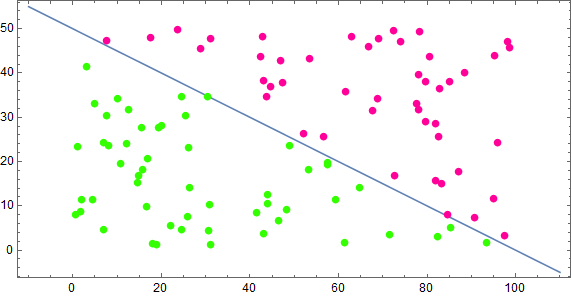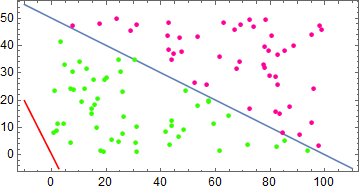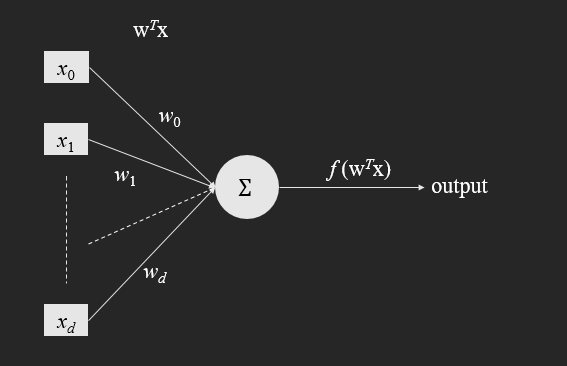Fenrier Lab

# 感知器

### 线性分类观点

$\omega^T x + b = 0$

$r(x) = \frac{\omega^T}{\|\omega\|} x+ \frac b{\|\omega\|}$

$y_i r(x^{(i)}) < 0$

$J(\omega, b) = -\sum_{x^{(i)} \in \mathcal{E}} y_i r(x^{(i)})$

$\min_{\omega ,b} \quad J(\omega, b)$

$J(\omega, b) = -\sum_{x^{(i)}\in \mathcal{E}} y_i \left(\frac {\omega^T}{\|\omega\|}x^{(i)} + \frac b {\|\omega\|}\right)\\ \Rightarrow \|\omega\|J(\omega, b) = -\sum_{x^{(i)}\in \mathcal{E}} y_i (\omega^T x^{(i)} + b)$

\begin{aligned} &\nabla_{\omega}(\sqrt{\omega^T \omega}) J + \sqrt{\omega^T \omega}\nabla_{\omega} J = -\sum_{x^{(i)}\in \mathcal{E}}y_i x^{(i)}\\ \Rightarrow& J\frac{\omega}{\|\omega\|}+ \|\omega\| \nabla_{\omega} J = -\sum_{x^{(i)}\in \mathcal{E}}y_i x^{(i)}\\ \Rightarrow&\nabla_{\omega} J = \frac 1 {\|\omega\|^2}\left(-\|\omega\|\sum_{x^{(i)}\in \mathcal{E}}y_i x^{(i)} -J \omega \right)\\ &= \frac 1 {\|\omega\|^2}\left(-\|\omega\|\sum_{x^{(i)}\in \mathcal{E}}y_i x^{(i)} + \sum_{x^{(i)}\in \mathcal{E}} y_i \left(\frac {\omega^T\omega} {\|\omega\|}x^{(i)} + \frac {b \omega} {\|\omega\|}\right) \right)\\ &=\frac {b\omega} {\|\omega\|^3}\sum_{x^{(i)}\in \mathcal{E}}y_i \end{aligned}

$\omega^{(k+1)} = \omega^{(k)} -\alpha \frac {b^{(k)}\omega^{(k)}} {\|\omega^{(k)}\|^3}\sum_{x^{(i)}\in \mathcal{E}}y_i$

$\hat{r}(x) = \omega^T x + b$

$J(\omega, b) = -\sum_{x^{(i)} \in \mathcal{E}} y_i (\omega^T x^{(i)}+b)$

$\nabla_\omega J = - \sum_{x^{(i)} \in \mathcal{E}} y_i x^{(i)}$

$\mathbf{w}^T \mathbf{x} = 0$

$\min_{\mathbf{w}}\quad J(\mathbf{w})$

$\mathbf{w}^{(k+1)} = \mathbf{w}^{(k)} + \alpha \nabla_{\mathbf{w}} J(\mathbf{w^{(k)}})$

$\nabla_{\mathbf{w}} J(\mathbf{w}) = -\sum_{\mathbf{x}^{(i)} \in \mathcal{E}} y_i \mathbf{x}^{(i)}$

### 一个简单的实现

//定义直线方程
Function<double[], Double> strightLine = x -> 100 - x - 2*x;
//随机数生成器
Random random = new Random();
random.setSeed(123);

//这里将类别标签值放在每个数组的第三个索引位置
List<double[]> data = IntStream.rangeClosed(1, 100).boxed()
.map(i -> new double[]{random.nextDouble() * 100, random.nextDouble() * 50})
.map(p -> new double[]{p, p, strightLine.apply(p) > 0 ? 1 : -1})
.collect(Collectors.toList());//直线系数数组
final double[] w = {1, 1, 1};
//系数数组，用于存储每次更新后的值
final double[] w2 = new double;
//学习速率
final double alpha = 0.01;
//判断直线是否将点 x 正确分类
Function<double[], Boolean> isRight = x -> x * (w + w * x + w * x) > 0;
//判断梯度下降是否收敛，这里使用两次更新差的模作为衡量标准，阈值设为 0.01
final double epsilon = 0.01;
BiFunction<double[], double[], Boolean> isConverge = (before, after) -> {
double[] sub = {after - before, after - before, after - before};
return Math.sqrt(sub * sub + sub * sub + sub * sub) < epsilon;
};


do{
w2 = w;
w2 = w;
w2 = w;
double[] delta = data.stream()
.filter(isRight::apply)
.map(x -> new double[]{x * 1, x * x, x * x})
.reduce(new double[]{0, 0, 0}, (d1, d2) -> new double[]{d1 + d2, d1 + d2, d1 + d2});

w -= alpha * delta;
w -= alpha * delta;
w -= alpha * delta;
}while(!isConverge.apply(w, w2));


$\mathbf{w} = [-622.05\quad 6.20\quad12.23]$### 单层感知器f(x) = \left\{\begin{aligned} & -1\quad x < 0\\ &1 \quad \quad x > 0 \end{aligned} \right.